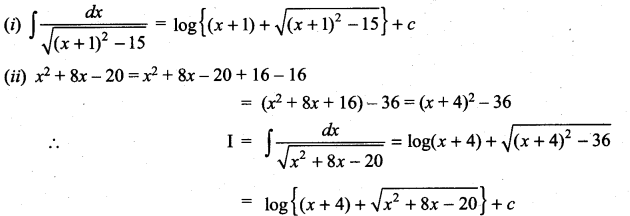# Samacheer Kalvi 11th Maths Solutions Chapter 11 Integral Calculus Ex 11.10

## Tamilnadu Samacheer Kalvi 11th Maths Solutions Chapter 11 Integral Calculus Ex 11.10

Find the integrals of the following
Question 1.
(i) $$\frac{1}{4-x^{2}}$$
(ii) $$\frac{1}{25-4 x^{2}}$$
(iii) $$\frac{1}{9 x^{2}-4}$$
Solution: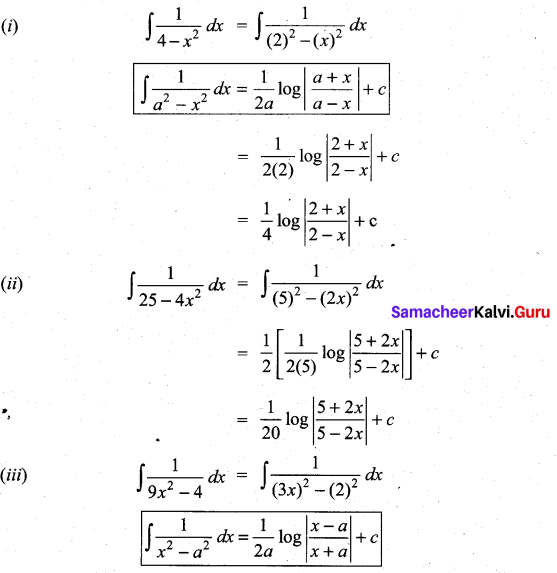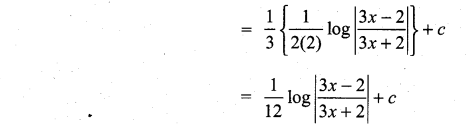Question 2.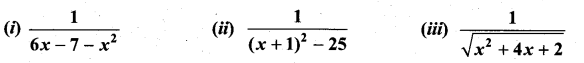Solution:
(i) Let I = $$\int \frac{1}{6 x-7-x^{2}} d x$$
Consider, -x2 + 6x – 7 = -[x2 – 6x + 4]
= -[(x – 3)2 – 9 + 7]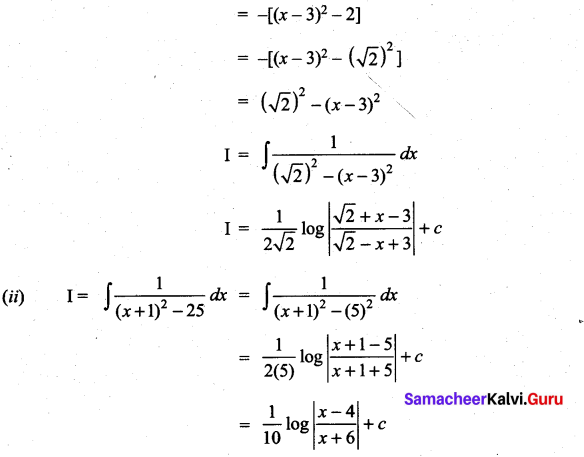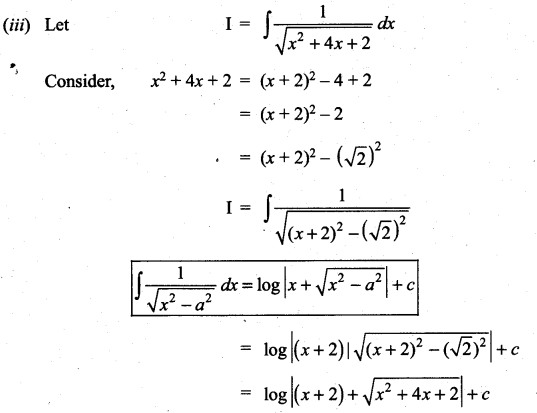Question 3.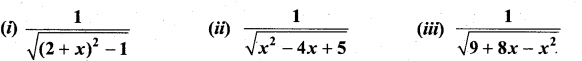Solution: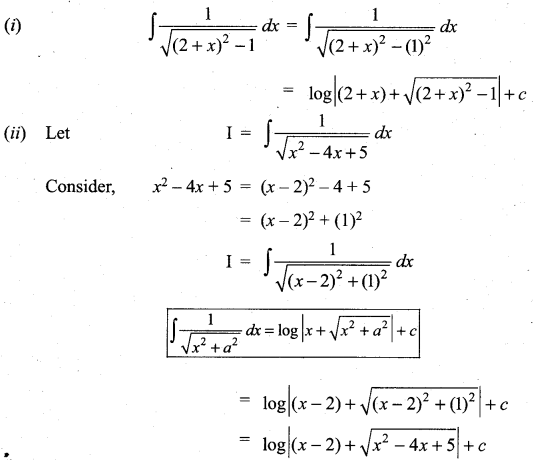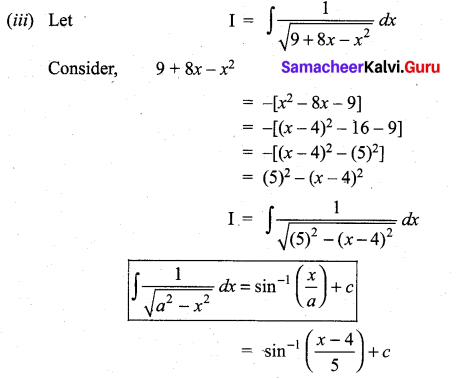### Samacheer Kalvi 11th Maths Solutions Chapter 11 Integral Calculus Ex 11.10 Additional Problems

Question 1.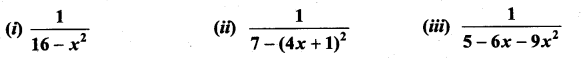Solution: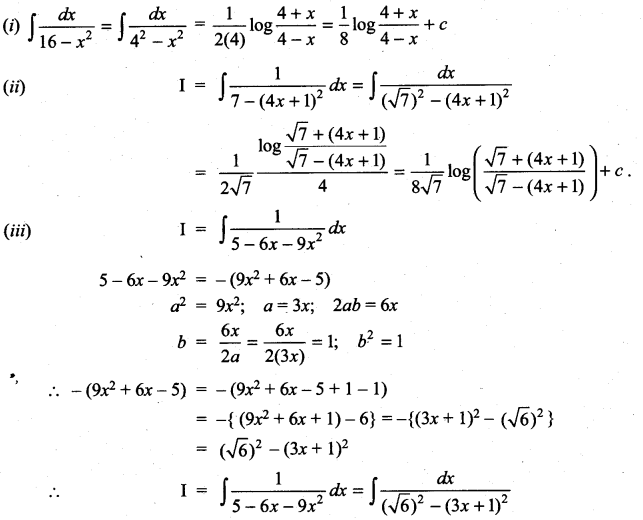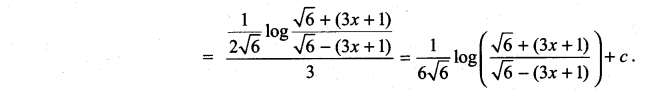Question 2.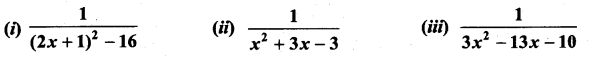Solution: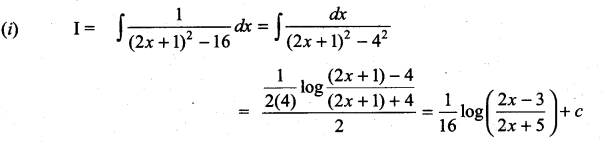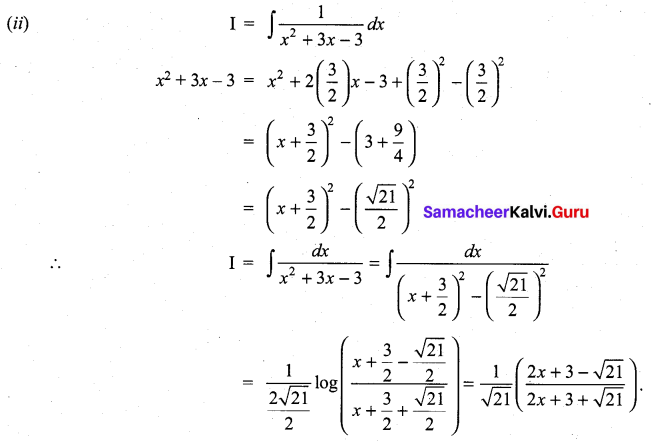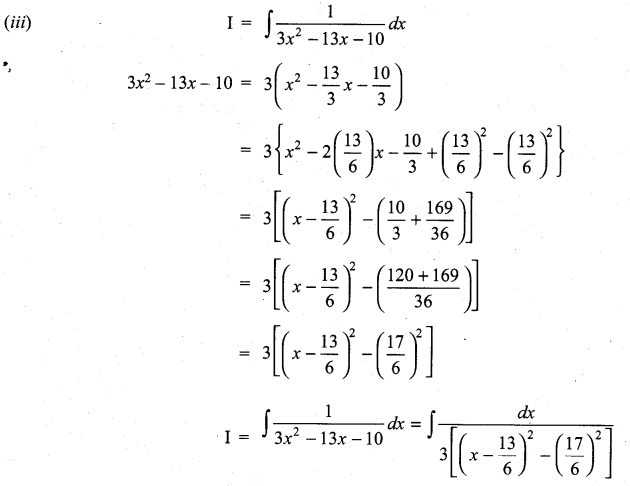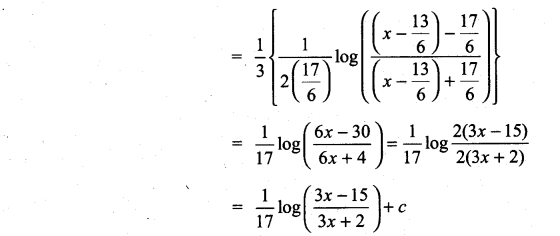Question 3.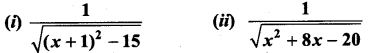Solution: